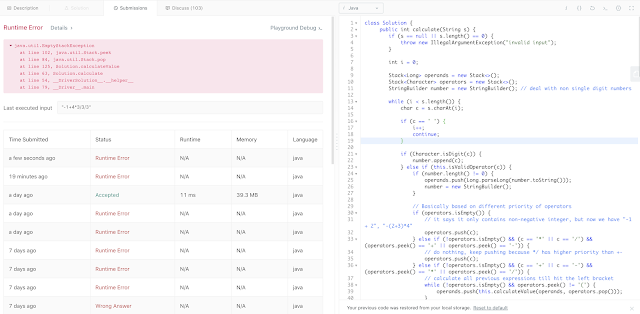## Sunday, August 18, 2019

### Problem Statement

Implement a basic calculator to evaluate a simple expression string.
The expression string may contain open `(` and closing parentheses `)`, the plus `+` or minus sign `-`non-negative integers and empty spaces .
The expression string contains only non-negative integers, `+``-``*``/` operators , open `(` and closing parentheses `)` and empty spaces . The integer division should truncate toward zero.
You may assume that the given expression is always valid. All intermediate results will be in the range of `[-2147483648, 2147483647]`.
Some examples:
```"1 + 1" = 2
" 6-4 / 2 " = 4
"2*(5+5*2)/3+(6/2+8)" = 21
"(2+6* 3+5- (3*14/7+2)*5)+3"=-12
```

Note: Do not use the `eval` built-in library function.

### Video Tutorial

You can find the detailed video tutorial here

### Thought Process

This is an extension problem to Basic Calculator and Basic Calculator II. The differences are this time it really looks like a real calculator where it can do "+-*/" and with brackets.

It's still the same thought process with exactly the same idea before: having two stacks,  one stack for the operator and the other for the operands, and with exactly the same calculating preferences before: calculate * and / before + and -. When seeing right bracket, continue popping till you see the left bracket.

This works fine assuming all the integers are non-negative, which they are supposed to be based on the problem description, and that's what most of the existing online leetcode solutions did as well.

As of 08/18/2019, this doesn't seem to be the case because leetcode decides to include "non-negative" integer test cases. See below case "-1+4*3/3/3"An "non-negative" integer case

Since we are practicing interviews, so let's also address the non-negative integer case as well

A few caveats
• (New) Handle negative integer starting case:
`"-1 + 2" or "-(2+3)*4"`
• (New) Handle negative integer in between expression case:
`"1 - (-7)"`
• (New) Calculate whenever you can calculate since division order matters
`15 / 3 / 5 should be 1, but it won't work 3 / 5 = 0,  then 15/0 invalid`
• Pay attention to the order when popping out operands and calculate, the order matters.
• The number might not be just single digit, so need to read the char and convert the numbers

### Solutions

#### Two stacks for non-negative integer solution

Keep two stacks, operator and operands as explained in the above "Thought Process"

Time Complexity: O(N), N is the length of the string
Space Complexity: O(N), extra stack is needed

#### Two stacks for negative integer solution

Essentially how do address the below two situations given the current code structure
• (New) Handle negative integer starting case. I simply just check if the first char in trimmed string is "-", and push a -1 into operands and "*" into operator. (e.g.,  -(a+b) = -1 * (a+b) and -a = -1 * a)
`"-1 + 2" or "-(2+3)*4"`
• (New) Handle negative integer in between expression case. This is a bit hacky because a  "-" in the middle of the expression could mean two things: a normal minus sign or a negative sigh denoting negative integer. Luckily "1 - - 2" would not be a valid case which means there should always be a left bracket before the negative sign for negative integer. What I did was using isNegativeNumberFollowingLeftBracket to find those cases and put a -1 and * into the operator and operands respectively
`"1 - (-7)"`

Time Complexity: O(N), N is the length of the string
Space Complexity: O(N), extra stack is needed